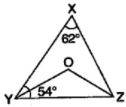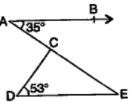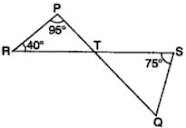# NCERT Solutions for Chapter 6 Lines and Angles Class 9 Maths

 Chapter Name NCERT Solutions for Chapter 6 Lines and Angles Class Class 9 Topics Covered Types of AnglesProperties of Angles Related Study Materials NCERT Solutions for Class 9 MathsNCERT Solutions for Class 9Revision Notes for Chapter 6 Lines and Angles Class 9 MathsImportant Questions for Chapter 6 Lines and Angles Class 9 MathsMCQ for for Chapter 6 Lines and Angles Class 9 Maths

## Short Revision for Ch 6 Lines and Angles Class 9 Maths

1. All the collinear points lie on the same line.
2. The inclination of two rays originating from the same end point is called the angle.
3. The rays making an angle are called the arms of the angle and the end point is called the vertex.
4. Types of angles: Acute angle, right angle, obtuse angle, straight angle, reflex angle etc.
5. An acute angle is greater than 0° but less than 90°.
6. A right angle is exactly equal to 90'.
7. An obtuse angle is greater than 90° but less than 180°.
8. A straight angle is exactly equal to 180°.
9. A reflex angle is greater than 180° but less than 360°.
10. The two angles whose sum is one right angle, i.e., 90°, are called complementary angles.
11. The complementary angle of an angle θ is 90° — θ.
12. The two angles whose sum is two right angles, i.e., 180°, are called supplementary angles.
13. The supplementary angle of an angle θ is 180° — θ.
14. If the sum of two adjacent angles is 180°, then they form a linear pair of angles.
15. If two lines intersect each other, then two pairs of vertically opposite angles are formed.
16. Vertically opposite angles are formed.
17. If a transversal intersects two parallel lines, then
(i) each pair of alternate interior angles is equal.
(ii) each pair of vertically opposite angles.
(iii) each pair of alternate exterior angles is equal.
(iv) each pair of corresponding angles is equal.
(v) sum of each pair of interior angles on the same side of the transversal is 180° .
18. Lines which are parallel to the same line are parallel to each other.
19. Lines which are perpendicular to the same line are parallel to each other.
20. The sum of the angles of a triangle 180°. This property is called Angle Sum Property of a triangle.
21. An exterior angle of a triangle is equal to the sum of the two interior opposite angles.

### Exercise 6.1

1. In Fig. 6.13, lines AB and CD intersect at O. If ∠AOC + BOE = 70° and BOD = 40°, find BOE and reflex COE.2. In Fig. 6.14, lines XY and MN intersect at O. If POY = 90° and a : b = 2 : 3, find c.3. In Fig. 6.15, PQR = PRQ, then prove that PQS = PRT.

5. In Fig. 6.17, POQ is a line. Ray OR is perpendicular to line PQ. OS is another ray lying between rays OP and OR. Prove that ROS = ½ (QOS – POS).6. It is given that XYZ = 64° and XY is produced to point P. Draw a figure from the given information. If ray YQ bisects ZYP, find XYQ and reflex QYP.

### Exercise: 6.2

1. In Fig. 6.28, find the values of x and y and then show that AB॥ CD.

2. In Fig. 6.29, if AB॥CD, CD॥EF and y : z = 3 : 7, find x.

3. In Fig. 6.30, if AB॥CD, EF ⊥ CD and GED = 126°, find AGE, GEF and FGE.

4. In Fig. 6.31, if PQ॥ ST, PQR = 110° and RST = 130°, find QRS.

[Hint : Draw a line parallel to ST through point R.]

5. In Fig. 6.32, if AB॥ CD, APQ = 50° and PRD = 127°, find x and y.6. In Fig. 6.33, PQ and RS are two mirrors placed parallel to each other. An incident ray AB strikes the mirror PQ at B, the reflected ray moves along the path BC and strikes the mirror RS at C and again reflects back along CD. Prove that AB॥ CD.### Exercise 6.3

1. In Fig. 6.39, sides QP and RQ of ΔPQR are produced to points S and T respectively. If SPR = 135° and PQT = 110°, find PRQ.

2. In Fig. 6.40, X = 62°, XYZ = 54°. If YO and ZO are the bisectors of XYZ and XZY respectively of Δ XYZ, find OZY and YOZ.3. In Fig. 6.41, if AB॥ DE, BAC = 35° and CDE = 53°, find DCE.4. In Fig. 6.42, if lines PQ and RS intersect at point T, such that PRT = 40°, RPT = 95° and TSQ = 75°, find SQT.